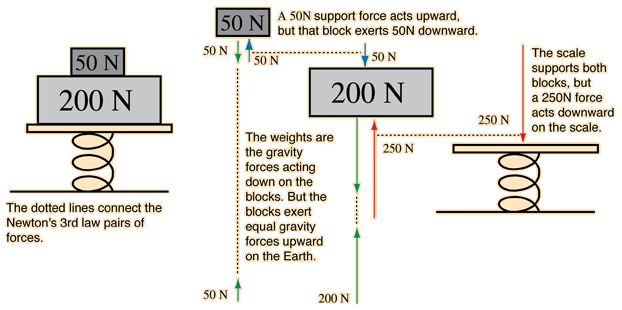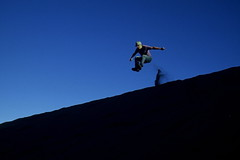Home » Posts tagged 'force'

# Tag Archives: force

## Friction – Numerical Problem

A block of mass 1 kg lies on a horizontal surface in a truck . The coefficient of static friction between the block and the surface is 0.6 .if the acceleration of the truct is 5m/s² calculate the frictional force acting on the block.Asked  Mrinmoy Das

## Numericals from Newton’s Laws of motion based on F=ma (For calss XI students of Kendriya Vidyalaya Pattom)

Download the questions and solve them and submit on or before 9 Oct 2014

1. A force acts for 10 s on a body of mass 10 kg after which the force ceases and the body describes 50 m in the next 5 s. Find the magnitude of the force. [Ans: 10 N]
2. A  truck starts from rest and rolls down a hill with constant acceleration. It travels a distance od 400 m in 20s. Calculate the acceleration and the force acting on it if its mass is 7 metric tonnes. [Ans: 2 m/s2, 14000N]
3. A motor car running aat the rate of 7 m/s can be stopped by applying brakes in 10 m. Show that total resistance to the motion, when the brakes are on is one fourth of the weight of the car.
4. In an Xray machine, an electron is subjected top a force of 10-23 N. In how much time the electron will cover a distance of 0.1 m,? Take the mass of the electron = 10-30 kg

## Numericals from Newton’s Laws of motion based on F=ma (For calss XI students of Kendriya Vidyalaya Pattom)

Download the questions and solve them and submit on or before 9 Oct 2014

1. A force acts for 10 s on a body of mass 10 kg after which the force ceases and the body describes 50 m in the next 5 s. Find the magnitude of the force. [Ans: 10 N]
2. A  truck starts from rest and rolls down a hill with constant acceleration. It travels a distance od 400 m in 20s. Calculate the acceleration and the force acting on it if its mass is 7 metric tonnes. [Ans: 2 m/s2, 14000N]
3. A motor car running aat the rate of 7 m/s can be stopped by applying brakes in 10 m. Show that total resistance to the motion, when the brakes are on is one fourth of the weight of the car.
4. In an Xray machine, an electron is subjected top a force of 10-23 N. In how much time the electron will cover a distance of 0.1 m,? Take the mass of the electron = 10-30 kg

## Force and acceleration – Can force be exerted without acceleration?

I am not able to understand this and I couldn’t think of a better example. i repeat. its just an example. if i punch someone’s face with my arm having constant velocity, it will mean that the acceleration of my arm is zero. F=ma, therefore force exerted by my arm is zero. Now if the force applied is zero the why does the person whom i punched experiences pain….. please help… thanks…It’s not whether your hand is moving with constant velocity, bu what happens to your hand after hitting the face of the opponent. The face resists the motion of your hand the momentum of your hand is imparted/shared to the face. And there is (-)acceleration to the hand and causes a force to be exerted on the face. The momentum imparted to the face will be responsible for the damage caused.## Does Newton’s Third Law apply on throwing butter on a wall?

Newton’s third law of motion states that

“When we throw any object on anything, that thing also pushes back with the same force. But when we throw butter on the wall, it sticks on the wall. Why?”

Asked by Akshit and Koushal from Class VII A from Kendriya Vidyalaya Air Force Station Bidar, Karnataka

I feel that there is some misconception/misunderstanding here.Newton’s Third law states that whenever two bodies interact each other, the force exerted by one body on the other is equal and opposite to the force exerted by the second body on first. These forces are simultaneous and are exerted on different bodies. tha is; the force exerted by the first body is acting on the second body and the force exerted by the second body is acted on the first body.

It doesn’t matter whether the body sticks to the other body or bounces back. We are concerned here with force and not with the motion.

When handful of butter (or clay) is thrown on to the wall, the ball of butter exerts some force on the wall. The wall exerts an equal force simultaneously. But the adhesive force between the wall and butter is more than the force acting on butter which tries to detach it. So it is not detached.When a rubber ball thrown on the wall, the force exerted by the wall on the ball compresses it. Due to the elastic nature of the ball, it tries to bring the shape back to original and this makes it bounce back. The force exerted on the wall due to the bouncing ball is double that of a clay/butter ball of same mass hitting the the wall with same speed; because, the rubber ball requires the force to bounce back too.

http://www.phy-astr.gsu.edu/dhamala/Physics2211/Chapter9.pdf## What is Pseudo force?

What is Pseudo force? Asked Rahul Answer: The word pseudo means false. Remmeber pseudopodia, the false legs of amoeba.(Also called fictitious force,or phantom force) An additional force due to nonuniform relative motion of two reference frames is called a pseudo-force. A pseudoforce does not arise from any physical interaction between two objects, but rather from the acceleration a of the non-inertial reference frame itself. A fictitious force on an object arises when the frame of reference used to describe the object’s motion is accelerating compared to a non-accelerating frame.## Why is it necessary to bend knees while jumping from a height?

Why is it necessary to bend knees while jumping from a height?

This is a consequence of the impulse momentum principle.When we bent our knees on landing, the time of impact increases and reduces the average force exerted during the entire process. This prevents the chance of damage to our body.

Impulse momentum principle states that the impulse of a force is equal to the change in momentum produced.

Impulse is measured as the product of force and time interval. So, when we stop       ourselves, there is a fixed change in momentum. If we stop ourselves very fast, the time interval is less and the average force will be very large.

But, if we bent our knees during landing, the time involved in the proces of landing will be more and thus the average force will be less and hence the damage will also be less.### Hits so far @ AskPhysics

• 2,274,296 hits